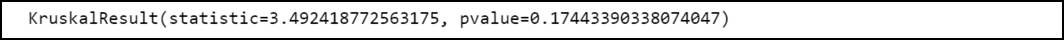GFG App
Open AppBrowser
Continue

# How to Perform a Kruskal-Wallis Test in Python

Kruskal-Wallis test is a non-parametric test and an alternative to One-Way Anova. By non-parametric we mean, the data is not assumed to become from a particular distribution. The main objective of this test is used to determine whether there is a statistical difference between the medians of at least three independent groups.

## Hypothesis:

The Kruskal-Wallis Test has the null and alternative hypotheses as discussed below:

• The null hypothesis (H0): The median is the same for all the data groups.
• The alternative hypothesis: (Ha): The median is not equal for all the data groups.

## Stepwise Implementation:

Let us consider an example in which the Research and Development team wants to determine if applying three different engine oils leads to the difference in the mileage of cars. The team decided to opt for 15 cars of the same brand and break down them into groups of three (5 cars in each group). Now each group is doped with exactly one engine oil (all three engine oils are used). Then they are allowed to run for 20 kilometers on the same track and once their journey gets ended, the mileage was noted down.

Step 1: Create the data

The very first step is to create data. We need to create three arrays that can hold cars’ mileage (one for each group).

## Python3

 `data_group1 ``=` `[``7``, ``9``, ``12``, ``15``, ``21``]` `data_group2 ``=` `[``5``, ``8``, ``14``, ``13``, ``25``]` `data_group3 ``=` `[``6``, ``8``, ``8``, ``9``, ``5``]`

Step 2: Perform the Kruskal-Wallis Test

Python provides us kruskal() function from the scipy.stats library using which we can conduct the Kruskal-Wallis test in Python easily.

## Python3

 `# Import libraries` `from` `scipy ``import` `stats`   `# Defining data groups` `data_group1 ``=` `[``7``, ``9``, ``12``, ``15``, ``21``]` `data_group2 ``=` `[``5``, ``8``, ``14``, ``13``, ``25``]` `data_group3 ``=` `[``6``, ``8``, ``8``, ``9``, ``5``]`   `# Conduct the Kruskal-Wallis Test ` `result ``=` `stats.kruskal(data_group1, data_group2, data_group3)`   `# Print the result` `print``(result)`

Output:Step 3: Analyze the results.

In this example, the test statistic comes out to be equal to 3.492 and the corresponding p-value is 0.174. As the p-value is not less than 0.05, we cannot reject the null hypothesis that the median mileage of cars is the same for all three groups. Hence, We don’t have sufficient proof to claim that the different types of engine oils used to lead to statistically significant differences in the mileage of cars.

My Personal Notes arrow_drop_up